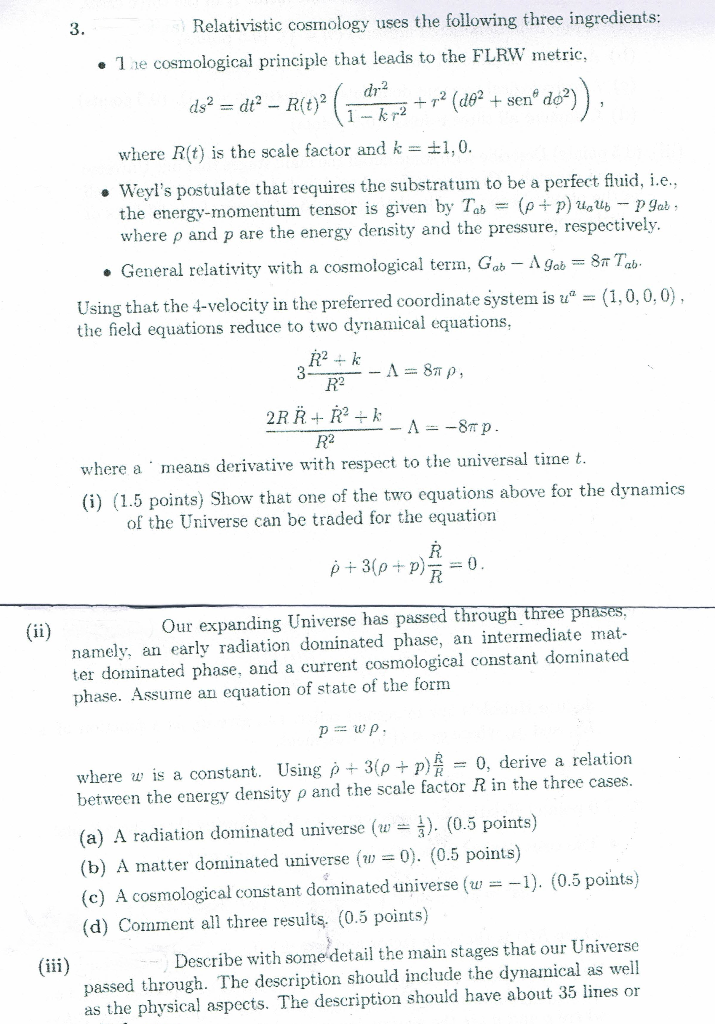# Solved: Relativistic Cosmology Uses The Following Three Ingredients: 3 1e Cosmological Principle That Leads To The FLRW Metric, Dr2 Ds2 = Dt2- R(t)2 1k Rde Sen Do Where R(t Is The Scale Factor And K =

By |Relativistic cosmology uses the following three ingredients: 3 1e cosmological principle that leads to the FLRW metric, dr2 ds2 = dt2- R(t)2 1k rde sen do where R(t is the scale factor and k = 1,0. Weyl's postulate that requires the substratum to be a perfect fluid, i.e. the energy-momentum tensor is given by Tab = (p p) uaub p gab : where p and p are the energy density and the pressure. respectively General relativity with a cosmological term. Gab - A gab = 8# Tab- (1,0,0,0 Using that the 4-velocity in the preferred coordinate system is u" = the field equations reduce to two dynamical equations 3 R2 2RRR2 -A-8m p R2 where a means derivative with respect to the universal time t (i) (1.5 points) Show that one of the two equations above for the dynamics of the Universe can be traded for the equation p3(p+p) =0 Our expanding Universe has passed through three phases., (ii) namely, an early radiation dominated phase, an intermediate mat- ter dominated phase, and a current cosmological constant dominated phase. Assume an equation of state of the form pwp where is a constant. Using p + 3(p + p) between the energy density p and the scale factor R in the three cases = 0, derive a relation )(0.5 points) (a) A radiation dominated universe (w (b) A matter dominated universe w0. (0.5 points) (c) A cosmological constant dominated universe (w = -1). (0.5 points) (d) Comment all three results. (0.5 points) Describe with some detail the main stages that our Universe (iii passed through. The description should include the dynamical as well as the physical aspects. The description should have about 35 lines or